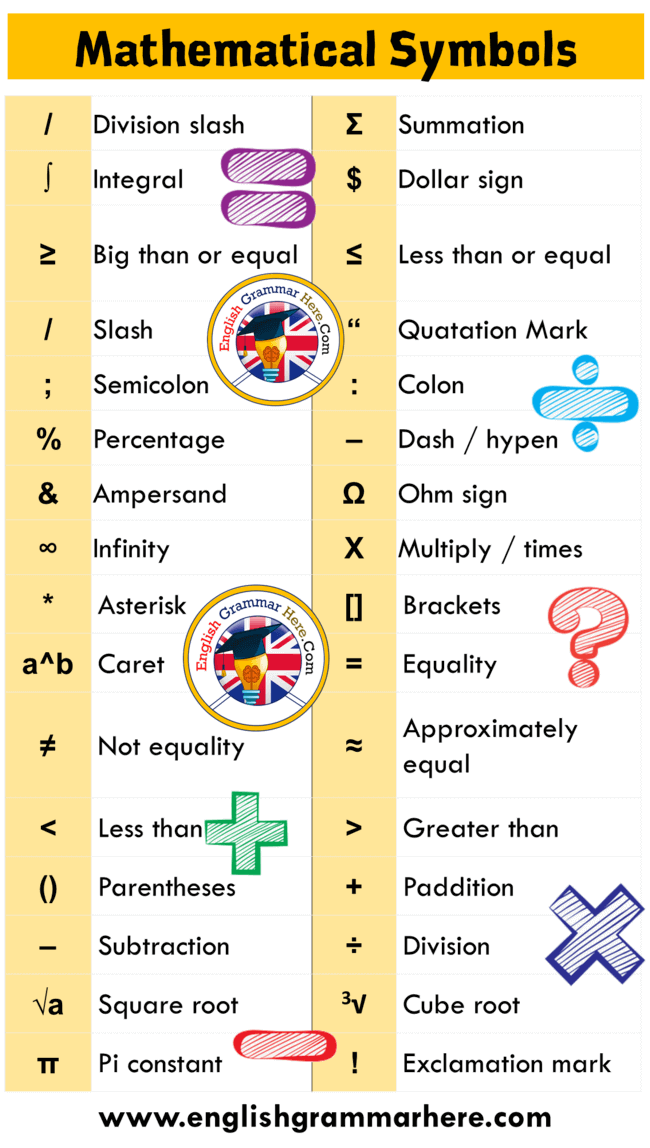# Mathematical Symbols Examples and Their Meanings

Mathematical Symbols Examples and Their Meanings#### Mathematical Symbols

In some universities, all courses are taught in English. Particularly, individuals who study in English at the university may have a lot of difficulty in understanding different subjects. In this case, individuals need to learn some terms in order to get high points from these courses. Today, we will examine the meanings of some mathematical symbols that will work for you both at high school and at university, and their English equivalents. You can note and repeat the ones you have difficulty in memorizing.

Here are the most used math symbols in the English language and their detailed meanings!

#### Mathematical Symbols Examples and Their Meanings

= equals sign

When you add a collection, you must use equals sign in between. : This sign gives information about the fact that two different variables have equal value with each other.

≠ equal not equal sign

I read the test paper but I couldn’t give you full points due to the not equal sign you used. : If you want to indicate that any transaction is not equal to the specified equivalence, you can also use the not equal sign.

≈ approximately equal

Instead of typing the comma after the comma long, you can specify the approximate values using the equal sign. Usually such signs can be used to indicate that values such as 3.8 are approximately equal to 4.

> strict inequality

You can create the meaning of hierarchy by using the strict inequality sign from different natural numbers and integers in order. Strict inequality is used to express that one number is larger than the other. For example, when we say 3> 2, we state that 3 is greater than 2.

< strict inequality

You can use the strict inequality sign to give less than meaning when comparing mathematical equations. This sign indicates that one value is smaller than another. For example, 5 <7.

≥ inequality

We do not always have to mention one possibility in mathematics, sometimes inequality and equality can coexist. When we want to indicate that one value is equal to or greater than another, we use the inequality sign. For example, N (set of natural numbers) ≥ 0.

≥ inequality

We can use inaquality symbols in mathematics to indicate a hierarchy in subjects such as equivalence. When we use the ≥ sign, we state that one variable can be equal to or greater than the other. For example, let’s talk about a set of positive numbers. Let’s name the set of positive numbers as A. In this case, A ≥ 1.

( ) parentheses

In order to always get the correct results in the language of mathematics, we have to enclose some operations in parentheses. Operations in parentheses in mathematics are calculated first within the framework of the transaction priority rule. Such rules help find the correct mathematical results. For example, in an operation such as 3 x (5 + 2), first add up and then multiply.

+ plus sign

We usually teach students the meaning of simple mathematical expressions like the plus sign in the primary school. In math, the plus sign demands that two different variables be added. Variables in different categories cannot be summed together. For example, 3 + 2 = 5.

– minus sign

Understanding simple mathematical symbols such as the minus sign actually means learning the basics of mathematics. When two different variables need to be subtracted from each other in math, the minus sign is usually used. For example, when you see a transaction like 3 – 2, you have to subtract 2 from the number 3 and the answer should be 1.

÷ division sign / obelus

Usually in mathematics education at the primary school level, it is necessary to focus on the obelus symbol after addition and subtraction and add the division to the curriculum. The division sign is used to find out how many times two variables are within each other. For example, it can be calculated as 9 ÷ 3 = 3.

% percent

Understanding the percent symbol, which you will often encounter in courses such as economics or economics, is really important to be successful at the university. The Percent symbol is used to denote the ratio of a given value to 100. For example, 20% ensures that 20 units are expressed in 100 units.

∥ parallel

It is important to know the parallel sign in order to understand the position of different shapes in space, usually in geometry lessons. The parallel sign is used to express that two different lines travel forever, never crossing each other. For example, it is possible to create a representation for lines A and B, such as A ∥